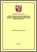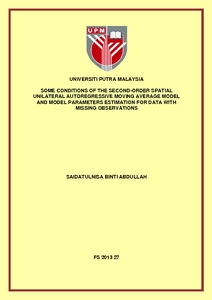# Some conditions of the second-order spatial unilateral autoregressive moving average model and model parameters estimation for data with missing observations

## Citation

Abdullah, Saidatulnisa (2013) Some conditions of the second-order spatial unilateral autoregressive moving average model and model parameters estimation for data with missing observations. PhD thesis, Universiti Putra Malaysia.

## Abstract

Spatial statistics has been given considerable attention in the past four decades. The primary purpose of such studies is for the benefit of modelling, forecasting and interpolation. The models studied however are first-order spatial models. In this thesis, we focus on the second-order spatial unilateral Autoregressive Moving Average model, the ARMA (2,2;2,2) model. The limitations of statistical models are based on the assumption that the model is stationary. In the early part of this thesis, we provide stationary conditions and their proofs for the spatial model chosen. The simulation results provide illustrations of generated stationary data when the stationary conditions are adhered to. We also provide illustrations when stationary conditions are violated. For the estimation of model parameters, the correlation structure for the model need to be developed. For the derivation of the explicit correlation structure this thesis will provide some initial terms by focussing on the sector of the fourth quadrant. Missing observations in a set of data raise problems for the analysis of spatial data sets. Missing data result in reduced sample size, lower statistical power and an increase in bias in the estimates. In this thesis, we introduce a method to handle this problem. This approach first uses available data to estimate the model parameters. A linear combination of backcast and forecast with information of the estimated parameters in the first step are then used to interpolate missing values. The Yule-Walker technique is utilized for the final model parameter estimation. Comparison of the absolute bias and the root mean square errors of the estimated parameters in simulation studies for complete data and incomplete data show significantly that the suggested technique can be suitably used for missing data with larger grid sizes and a lower percentage of data missing. The bias and root mean square error, however, tend to increase with smaller grid size and a higher percentage of data missing. Finally, some suggestions are given for further research for the properties of the ARMA (2,2;2,2) model especially in the area of the derivation of initial terms to enable model fitting using real data. For parameter estimation in the problem of missing observations in spatial data, techniques using other estimators can be developed and its performance compared with the YW estimator.Preview
PDF
FS 2013 27 IR.pdfView Item